#### Dictionary of Options

This section describes the EFFECTPLOT options in alphabetical order.

ALPHA=value

specifies the significance level,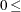value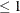, for producing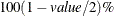prediction and confidence limits. By default, value=0.05.

AT <contopt> <classopt> <variable1=varopt <variable2=varopt…>>
where contopt= MEAN | MIN | MAX | MIDRANGE
classopt= ALL | REF
varopt= contopts | number-list |  classopts | ’class-level’…’class-level’

specifies values at which to fix continuous and class variables when they are not used in X=, Y=, SLICEBY=, or PLOTBY= effects. The contopt keyword fixes continuous variables at their mean, minimum, maximum, or midrange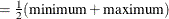; the default is to use the mean. The classopt keyword either fixes a CLASS variable at its reference (last) level or indicates that all levels of the CLASS variable should be processed; the default is to use the reference level. The varopt values enable you to specify contopt and classopt keywords, or to specify lists of numbers or class levels. You can specify a CLASS variable only once in the AT specification, but you can specify a continuous variable multiple times; for example, the following syntax is valid when X is a continuous variable:

effectplot / at(x=min max x=0 to 2 by 1 x=2 5 7);


Duplicate AT values are suppressed, so the last X=2 value is ignored.

You can also specify plug-in values for CLASS variable levels when computing the predicted values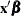. For example, suppose a CLASS variable A with two levels={0,1} is in the model. Then instead of using the coding for A in thevector by specifying AT(A=all), AT(A=ref) or AT(A=’0’ ’1’), you can specify a numeric list to plug in. For example, if the proportion of A’s that equal 0 in the data set is 0.3, then you can input the proportions for all levels of the variable by specifying AT(A=0.3 0.7). Under GLM coding, A=0 is coded as 1 0 and A=1 is coded as 0 1, so the plug-in specification replaces both of these codings with 0.3 0.7. Under REFERENCE coding A=0 is coded as 1 and A=1 is coded as 0, so this specification replaces both of these codings with 0.3 followed by 0.7; however, if another variable is nested within A, then only 0.3 is used. To plug in values, you must specify a multiple of the number of parameters used for the CLASS variable or, if a variable is nested within the CLASS variable, a multiple of the number of levels of the CLASS variable.

The plug-in values are distributed through the rest of the model effects in the following fashion. If a variable is nested within a plug-in variable, then its coding is multiplied by the plug-in value for the level it is nested in. If a variable interacts with a plug-in variable, its coding is multiplied by the appropriate plug-in value for the level it is interacting with. Lag, multimember, polynomial, and spline constructed effects are affected only by interactions and nestings. If the plug-in variable is part of a collection effect, then its values are replaced by the plug-in values; collection effects are also affected by interactions and nestings.

The AT levels are used for computing the predicted values. If the OBS option is also specified, then all observations are still displayed on all of the plots. For example, if you specify the options AT(A=’1’) OBS, then the fitted values are computed with A=1, but all of the observations are displayed with their predicted values computed at their observed level of A. If you want to display only a subset of the observations based on the levels of a CLASS variable, then you must specify either the PLOTBY= option or the OBS(BYAT) option.

ATLEN=n

specifies the maximum length (1n256) of the levels of the AT variables that are displayed in footnotes and headers. By default, up to 256 characters of the CLASS levels are displayed, and the continuous AT levels are displayed with a BEST format that has a width greater than or equal to 5, which distinguishes each level. Caution: If the levels of your AT variables are not unique when the first n characters are displayed, then the levels are combined in the plots but not in the underlying computations. Also, at most n characters for continuous AT variables are displayed.

ATORDER=ASCENDING | DESCENDING

uses the AT values for continuous variables in ascending or descending order as specified. By default, values are used in the order of their first appearance in the AT option.

CLI

displays normal (Wald) prediction limits. This option is available only for normal distributions with identity links. If your model is from a Bayesian analysis, then sampling-based intervals are computed; see the section Analysis Based on Posterior Estimates in Chapter 69: The PLM Procedure, for more information.

CLM

displays confidence limits. These are computed as the normal (Wald) confidence limits for the linear predictor, and if the ILINK option is specified, the limits are also back-transformed by the inverse link function. If your model is from a Bayesian analysis, then sampling-based intervals are computed; see the section Analysis Based on Posterior Estimates in Chapter 69: The PLM Procedure, for more information.

CLUSTER<=percent>

modifies the BOX and INTERACTION plot-types by displaying the levels of the SLICEBY= effect in a side-by-side fashion. You can specify percent as a percentage of half the distance between X levels. The percent value must be between 0.1 and 1; the default percent depends on the number of X levels, the number of SLICEBY levels, and the number of PLOTBY levels for INTERACTION plot-types. Default clustering can be removed by specifying the NOCLUSTER option.

CONNECT

modifies the BOX and INTERACTION plot-types by connecting the predicted values with a line. Default connecting lines can be removed by specifying the NOCONNECT option.

EXTEND=DATA | value

extends continuous covariate axes by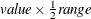in both directions, where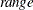is the range of the X axis. Specifying the DATA keyword displays curves to the range of the data within the appropriate SLICEBY=, PLOTBY=, and AT level. For the CONTOUR plot-type, value=0.05 by default; other plot-types set the default value to 0. When constructed effects are present, only the EXTEND=DATA option is available.

GRIDSIZE=n

specifies the resolution of curves by computing the predicted values at n equally spaced x-values and specifies the resolution of surfaces by computing the predicted values on an nn grid of points. Default values are n = 200 for curves and bands, n = 50 for surfaces, and n = 2 for lines. If results of a Bayesian or bootstrap analysis are being displayed, then the defaults are n = 500000/B, where B is the number of samples, the upper limit is equal to the usual defaults, and the lower limit equal to 20.

displays the fit on the scale of the inverse link function. In particular, the results are displayed on the probability scale for logistic regression. By default, a procedure displays the fit on either the link or inverse link scale.

INDIVIDUAL

displays individual probabilities for polytomous response models with cumulative links on the scale of the inverse link function. This option is not available when the LINK option is specified, and confidence limits are not available with this option.

LIMITS

invokes the CLI and CLM options.

displays the fit on the scale of the link function; that is, the linear predictor. Note that probabilities or observed proportions near 0 and 1 are transformed to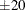. By default, a procedure displays the fit on either the link or inverse link scale.

MOFF

moves the offset for a Poisson regression model to the response side of the equation. If the ILINK option is also in effect, then the rate is displayed on the Y axis, while the LINK option displays the log of the rate on the Y axis. Without this option, the predicted values are computed and displayed only for the observations.

NCOLS=n

specifies the maximum number of columns in a paneled plot. This option is not available with the BOX plot-type.

The default choice of NROWS= and NCOLS= is based on the number of PLOTBY= and AT levels. If there is only one plot being displayed in a panel, then NROWS=1 and NCOLS=1 and the plots are produced as if you specified only the UNPACK option. If only two plots are displayed in a panel, then NROWS=1 and NCOLS=2. For all other cases, a 2x2, 2x3, or 3x3 panel is chosen based on how much of the last panel is used, with ties going to the larger panels. For example, if 14 plots are being created, then this requires either four 2x2 panels with 50% of the last panel filled, three 2x3 panels with 33% of the last panel filled, or two 3x3 panels with 55% of the last panel filled; in this case, the 3x3 panels are chosen.

If you specify both of the NROWS= and NCOLS= options, then those are the values used. However, if you only specify one of the options but have fewer plots, then the panel size is reduced; for example, if you specify NROWS=6 but only have four plots, then a plot with four rows and one column is produced.

NOCLI

suppresses the prediction limits.

NOCLM

suppresses the confidence limits.

NOCLUSTER

modifies the BOX and INTERACTION plot-types by preventing the side-by-side display of the levels of the SLICEBY= effect.

NOCONNECT

modifies the BOX and INTERACTION plot-types by suppressing the line that connects the predicted values.

NOLIMITS

invokes the NOCLI and NOCLM options.

NOOBS

suppresses the display of observations and overrides the specification of the OBS= option.

NROWS=n

specifies the maximum number of rows in a paneled plot. This option is not available with the BOX plot-type. See the NCOLS= option for more details.

OBS<(obs-options)>

displays observations on the effect plots. An input data set is required; hence the OBS option is not available with PROC PLM. The OBS option is overridden by the NOOBS option. When the ILINK option is specified with binary response variables, then either the observed proportions or a coded value of the response is displayed. For polytomous response variables, the observed values are overlaid onto the fitted curves unless the LOCATION= option is specified. Whether observations are displayed by default or not depends upon the procedure. If the PLOTBY= option is specified, then the observations displayed on each plot are from the corresponding PLOTBY= level for classification effects; for continuous effects, all observations are displayed on every plot.

The following obs-options are available:

BYAT

subsets the observations by AT level and by the PLOTBY= level. If you specify the PLOTBY= option without specifying this option, the observations are displayed on the plots that correspond to their PLOTBY= level without regard to any classification variables specified in the AT option. However, for FIT plot-types a distance can be computed and displayed (see the DISTANCE option for more information). This option is ignored when there are no AT variables.

controls the display of observations on contour plots. The keyword OUTLINE displays the observations as circles, GRADIENT displays gradient-colored dots, OUTLINEGRADIENT displays gradient-filled-circles, and NONE suppresses the display of the observations. The default is CDISPLAY=OUTLINEGRADIENT.

specifies what the gradient-shading of the observed values on the CONTOUR plot-type represents. The RESIDUAL keyword shades the observations by the raw residual value and displays the fitted surface as a line contour plot. The DEPENDENT keyword shades the observations by the response variable value and displays the fitted surface as a contour shaded on the same scale. The default is CGRADIENT=DEPENDENT.

DEPTH=depth

specifies the number of overlapping observations that can be distinguished by adjusting their transparency; you can specify 1depth100. By default, DEPTH=1. The DEPTH= option is available with FIT, SLICEFIT, and INTERACTION plot-types.

DISTANCE

displays observations on FIT plot-types with a color-gradient that indicates how far the observation is from the AT and PLOTBY= level. This option is ignored unless an AT or PLOTBY= option is specified.

The distance is computed as the square root of the following number: for each continuous AT and PLOTBY= variable, add the square of the difference from the observed value divided by the range of the variable; for each CLASS AT and PLOTBY= variable, add 1 if the CLASS levels are different. Thus the largest possible distance is the square root of the number of AT and PLOTBY= variables. Observations at zero distance are displayed with the darkest color, and the color fades as the distance increases.

Note that the UNPACKed panels compute the maximum distance within each panel and hence do not use the same gradient across all panels. Also, the PANELS panel-type computes the maximum distance within each PLOTBY= level, so a different gradient is used for each PLOTBY= level. All other panel-types compute the maximum distance across all observations and therefore use the same gradient on every plot.

FITATCLASS

computes fitted values only for class levels that are observed in the data set. This option is ignored when the GLM parameterization is used.

FRINGE

displays observations in a fringe (rug) plot at the bottom of the plot. This option is available only with FIT and SLICEFIT plot-types.

JITTER<(jitter-options)>

shifts (jitters) the observations. By default, the jittering in the X direction is achieved by adding a random number that is generated according to a normal distribution with mean=0 and standard deviation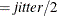and truncating at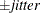, where jitter=0.01 times the range of the X axis; the jittering in the Y direction is performed independently but in the same fashion. The JITTER option is not available with the BOX plot-type. The following jitter-options are available:

FACTOR=factor

sets the jitter to factor times the range of the axis, and jitters in both the X and Y directions. You can specify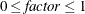.

SEED=seed

specifies an integer to use as the initial seed for the random number generator. If you do not specify a seed, or if you specify a value less than or equal to zero, then the time of day from the computer clock is used to generate an initial seed.

X=x-jitter

sets the jitter to x-jitter for the X direction; the jitter in the Y direction is assumed to be 0 unless the Y= option is also specified. You can specify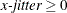. The X= option is not available for the INTERACTION plot-type. This option is ignored if the FACTOR= option is also specified.

Y=y-jitter

sets the jitter to y-jitter for the Y direction; the jitter in the X direction is assumed to be 0 unless the X= option is also specified. You can specify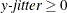. This option is ignored if the FACTOR= option is also specified.

LABEL<=OBS>

labels markers with their observation number.

LOCATION=location

specifies where the observed values for polytomous response models are displayed when the SLICEBY= variable is the response. This option is available only with the SLICEFIT and INTERACTION plot-types. The observations are always displayed at their appropriate X-axis value, but their Y-axis location can depend on the specification of the YRANGE= option or on the minimum and maximum computed predicted values in addition to the specified location. The following locations are available:

BOTTOM<=factor>

displays the first response level at the minimum predicted value, and displays succeeding response levels above the first level atintervals, whereis the range of the predicted values. You can specify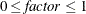, but the largest usable value, which corresponds to LOCATION=SPREAD, is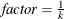, where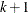is the number of response levels that are displayed. By default,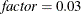.

CURVE

displays the observations for polytomous response models at their predicted values. For displays on the LINK scale, the reference level is displayed at the maximum value. This method is the default.

FIRST

displays the observations for a response level at the first displayed predicted value for that response level.

MAX

displays the observations for a response level at the maximum displayed predicted value for that response level.

MIDDLE

displays the observations for a response level at the middle of the displayed predicted values for that response level.

MIN

displays the observations for a response level at the minimum displayed predicted value for that response level.

displays the observations with the response levels evenly spread across the Y axis.

TOP<=factor>

displays the last response level at the maximum predicted value, and displays preceding response levels below the last level atintervals, whereis the range of the predicted values. You can specify, but the largest usable value, which corresponds to LOCATION=SPREAD, is, where k+1 is the number of response levels that are displayed. By default,.

PLOTBY<(panel-type)>=effect<=numeric-list>

specifies a variable or CLASS effect at whose levels the predicted values are computed and the plots are displayed. You can specify the response variable as the effect for polytomous response models. The panel-type argument specifies the method in which the plots are grouped for the display. The following panel-types are available.

COLUMNS

specifies that the columns within each panel correspond to different levels of the PLOTBY= effect and hence the rows correspond to different AT levels.

PACK

specifies that plots be displayed in the panels as they are produced with no control over the placement of the PLOTBY= and AT levels.

PANELS  | LEVELS

specifies that each level of the PLOTBY= effect begin a new panel of plots and the AT levels define the plots within the panels.

ROWS

specifies that the rows within each panel correspond to different levels of the PLOTBY= effect and hence the columns correspond to different AT levels.

This option is ignored with the BOX plot-type; box plots are always displayed in an unpacked fashion, grouped by the PLOTBY= and AT levels. If you specify a continuous variable as the effect, then you can either specify a numeric-list of values at which to display that variable or, by default, five equally spaced values from the minimum variable value to its maximum are displayed.

The default panel-type is based on the number of PLOTBY= and AT levels as shown in the following table.

Number of
PLOTBY Levels

Number of
AT Levels

Resulting
panel-type

1

1

(UNPACK)

>1

1

PACK

1

>1

PACK

2

>1

ROWS

3

>1

COLUMNS

>3

>1

PANELS

The default dimensions of the panels are also based on the number of PLOTBY= and AT levels; see the NCOLS= option for details.

Specification of the panel-type is honored except in the following cases. If you specify a panel-type but produce only one plot, specify the NROWS=1 and NCOLS=1 options, or specify the UNPACK option, then the plots are produced as if you specified only the UNPACK option. If you specify the PANELS panel-type with only one AT level, then the plots are produced with the UNPACK option. However, if you specify the PANELS panel-type but the PLOTBY= effect has only one level, then the panel-type is changed to PACK.

PLOTBYLEN=n

specifies the maximum length (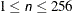) of the levels of the PLOTBY= variables, which are displayed in footnotes and headers. By default, up to 256 characters of the CLASS levels are displayed. Caution: If the levels of your PLOTBY= variables are not unique when the first n characters are displayed, then the levels are combined in the plots but not in the underlying computations.

POLYBAR

displays polytomous response data as a stacked histogram with bar heights defined by the individual predicted value. Your response variable must be the effect specified in the SLICEBY= option. If you specify the INDIVIDUAL option, then the histogram bars are displayed in a side-by-side fashion. If you specify the CLM option, then error bars are displayed on the side-by-side histogram bars.

PREDLABEL='label'

specifies a label to be displayed on the Y axis. The default Y axis label is determined by your model. For the CONTOUR plot-type, this option changes the title to label for Y.

SHOWCLEGEND

displays the gradient-legend for the CONTOUR plot-type. This option has no effect when the OBS(CGRADIENT=RESIDUAL) option is also specified.

SLICEBY=NONE | effect<=numeric-list>

displays the fitted values at the different levels of the specified variable or CLASS effect. You can specify the response variable as the effect for polytomous response models. Use this option to modify SLICEFIT, INTERACTION, and BOX plot-types. If you specify a continuous variable as the effect, then you can either specify a numeric-list of values at which to display that variable or, by default, five equally spaced values from the minimum variable value to its maximum are displayed. The NONE keyword is available for preventing the INTERACTION plot-type from slicing by a second class covariate. Note that the SLICEBY=NONE option is not available for the SLICEFIT plot-type, since that is the same as the FIT plot-type. The BOX plot-type accepts only classification effects.

SMOOTH

overlays a loess smooth on the FIT plot-type for models that have only one continuous predictor. This option is not available for binary or polytomous response models.

UNPACK

suppresses paneling. By default, multiple plots can appear in some output panels. Specify UNPACK to display each plot separately.

X=effect

specifies values to display on the X axis. For BOX and INTERACTION plot-types, effect can be a CLASS effect in the MODEL statement. For FIT, SLICEFIT, and CONTOUR plot-types, effect can be any continuous variable in the model.

Y=args

specifies values to display on the Y axis for the CONTOUR plot-type. The Y= argument can be any continuous variable in the model.

YRANGE=CLIP | (<min><,max>)

displays the predicted values on the Y axis in the range [min,max]. The YRANGE=CLIP option has the same effect as specifying the minimum predicted value as min and the maximum predicted value as max. The axis might extend beyond your specified values. By default, when the Y axis displays predicted probabilities, the entire Y axis, [0,1], is displayed. This option is useful if your predicted probabilities are all contained in some subset of this range. This option is not available with the CONTOUR plot-type.